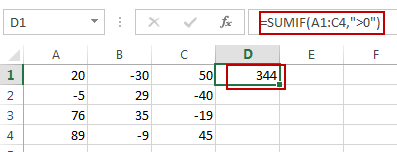# Sum Only Positive Numbers or Negative Numbers in Excel

This post will guide you how to sum only negative numbers in Excel. How to sum only positive numbers in Excel. How do I sum both positive and negative numbers in Excel. How to make the sum in a range show as a negative number in Excel.

## Sum Only Positive Numbers or Negative Numbers

If you want to sum only positive or negative numbers in excel, you can use a formula based on the SUMIF function.

For example, you have a range of cells (A1:C4) that contain negtive and positive numbers, and you want to sum only positive numbers, you can use the following formula:

`=SUMIF(A1:C4,">0")`

Type this formula into a blank cell and then press Enter key.

###If you want to sum only negative numbers in a given range of cells, you can use the following formula:

`=SUMIF(A1:C4,"<0")`

### Related Functions

• Excel SUMIF Function
The Excel SUMIF function sum the numbers in the range of cells that meet a single criteria that you specify. The syntax of the SUMIF function is as below:=SUMIF (range, criteria, [sum_range])…